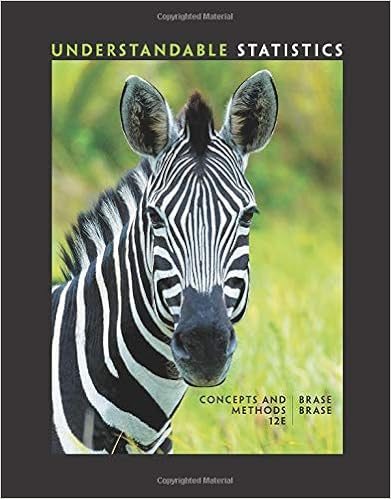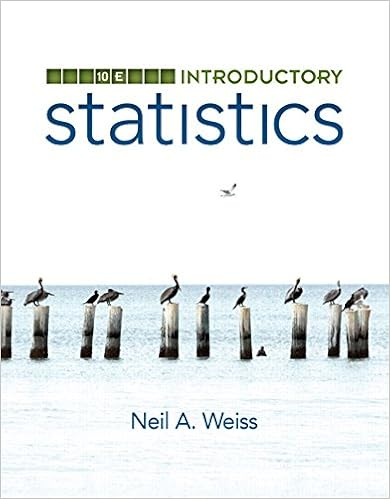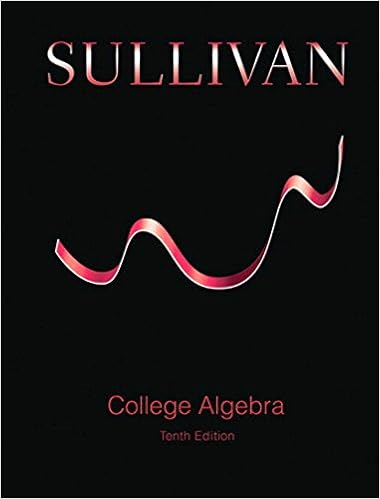# 10.1_review_a___c (2).doc - Name: 10.1A and C Review AP...

• Notes
• 2
• 100% (1) 1 out of 1 people found this document helpful

This preview shows page 1 - 2 out of 2 pages.

Name:AP Statistics10.1A and C ReviewSuppose you administer a certain aptitude test to a random sample of 9 students in your school, and that theaverage score is 105.We want to determine the mean µ of the population of all students in the school.Assumea standard deviation of  = 15 for the test.1.What is the upper critical value for a 98% confidence interval?(Show the key numbers in the sketch of thedistribution.)-3.03.0.50-.502.Explain the meaning of “98% confident.”3.What is the standard deviation of the mean,x4.Calculate a 98% confidence interval for the mean score µ for the whole school. Follow the InferenceToolbox.5.What sample size would be needed to have a margin of error at most 4 points??Chapter 10
##### We have textbook solutions for you!The document you are viewing contains questions related to this textbook.
Chapter 7 / Exercise 10
Understandable Statistics: Concepts and Methods
BraseExpert VerifiedBrowse all Textbook Solutions
From a population with standard deviation 25, a sample of size 100 is drawn.The mean of the sample is 235.1.Findz* for a 90% confidence interval for the true mean of the
End of preview. Want to read all 2 pages?

Course Hero member to access this document

Term
Fall
Professor
samford
##### We have textbook solutions for you!
The document you are viewing contains questions related to this textbook.The document you are viewing contains questions related to this textbook.
Chapter 7 / Exercise 10
Understandable Statistics: Concepts and Methods
BraseExpert Verified
•••